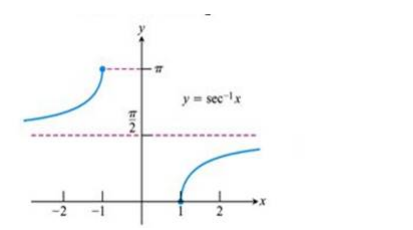# Mark the tick against the correct answer in the following:

Question:

Mark the tick against the correct answer in the following:

Domain of $\sec ^{-1} x$ is

A. $[-1,1]$

B. $R-\{0\}$

C. $R-[-1,1]$

D. $R-\{-1,1\}$

Solution:

To Find: The Domain of $\sec ^{-1}(x)$

Here,the inverse function is given by $y=\mathrm{f}^{-1}(x)$

The graph of the function $y=\sec ^{-1}(x)$ can be obtained from the graph of

$Y=\sec x$ by interchanging $x$ and $y$ axes.i.e, if $(a, b)$ is a point on $Y=\sec x$ then $(b, a)$ is the point on the function $y=\sec ^{-1}(x)$

Below is the Graph of the domain of $\sec ^{-1}(x)$From the graph, it is clear that the domain of $\sec ^{-1}(x)$ is a set of all real numbers excluding $-1$ and 1 i.e, $\mathrm{R}$ -

$[-1,1]$